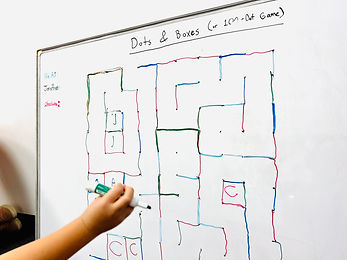## Ms. AJ

### Target 1​

###### Lesson Type:

Continuation

Data Probability

:

Probability

Understand probability as the possible outcomes of an event.

###### 1:

Understand that the likelihood of an event occurring can be described numerically and used to make predictions.

###### 2:

Classify events as certain, possible, or impossible.

###### 3:

Recognize that not all events occur with the same likelihood.

###### 4:

Define fair vs. unfair.

###### 5:

Identify the possible outcomes of an event.

5th

###### Vocabulary:

Probability, Chance, Outcome, Likely, Unlikely, Certain, Impossible, Random, Fair, Unfair

Activities:

Students worked on probability activities in their workbooks, reviewing what they had learned the previous week.

Students played with number & color spinners and identified different probabilities of the following: each color being landed on, even numbers being landed on, odd numbers being landed on.

Students did spin trials for even vs odd numbers and tallied their results. They compared their outcomes to their initial probabilities.

They then did dice trials with 10-sided dice, choosing 5 numbers for points (probability equaling 5 out of 10). They tested whether or not their probabilities matched their outcomes.

Students learned how probabilities can be written in fraction form, and how fractions can connect.### Home Exploration

###### Guiding Questions:## Absent Students:

Coco, Neha, Maverick

### Target 2

:

###### 1:

Use the given information in a problem to identify the correct answer.

###### 2:

Use the given information to determine which is not the correct answer.

###### 3:

Think outside of the box to solve problems.

###### 4:

Follow given rules to solve problems.

4th

###### Vocabulary:

Strategy

Activities:

Students played the game "Dots & Boxes" on a 100-dot grid. They were to take turns making a single horizontal or vertical line between dots, with the aim of creating squares. The goal is to determine strategies, particularly when the board starts to be filled up with lines. Where can they get the most boxes? How can they prevent others from getting too many?

Students reviewed the game "SET".### Home Exploration

###### Guiding Questions:### Target 3

:

###### Vocabulary:

Activities:### Home Exploration